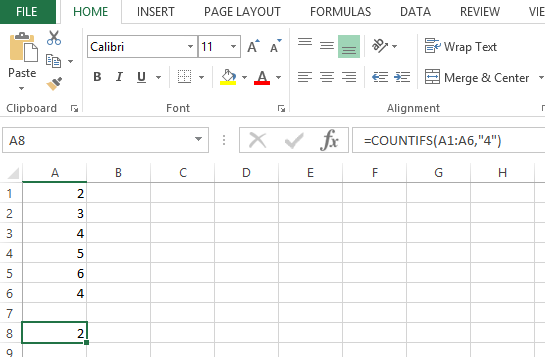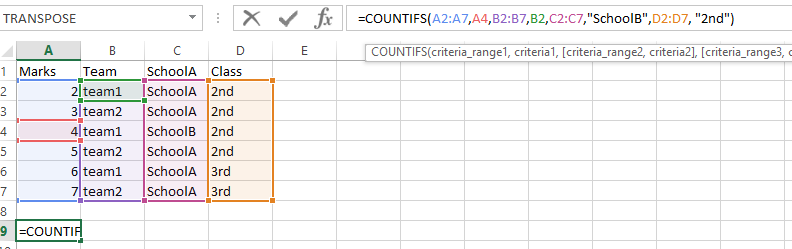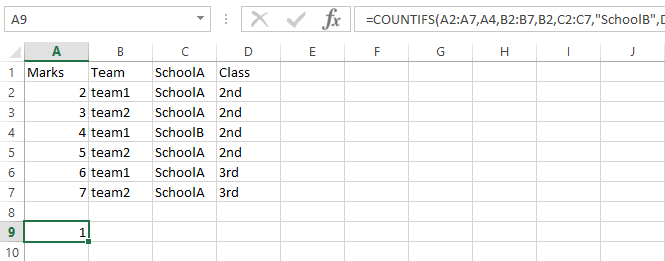### How To Use COUNTIFS Function

In this Article we will try to learn all the possible ways to use the COUNTIFS functions. Let us start with simple COUNTIFS function:

Lets us use simple data and use the formula:

=COUNTIFS(A1:A6,”4″)The result is 1 as it found simple instance of 4.But if we have more than one conditions in more than one ranges we will have to use the COUNTIFS instead of the simple countif excel function.

Let have the following data. Lets find someone from team1 with 4 marks:

=COUNTIFS(A2:A7,A4,B2:B7,B2)Lets see if we have more conditions. I’m adding more data.Let us see the result of the following formula: =COUNTIFS(A2:A7,A4,B2:B7,B2,C2:C7,”SchoolB”,D2:D7, “2nd”)Please find below the result: 1 (row 4)## Template

```Further reading: